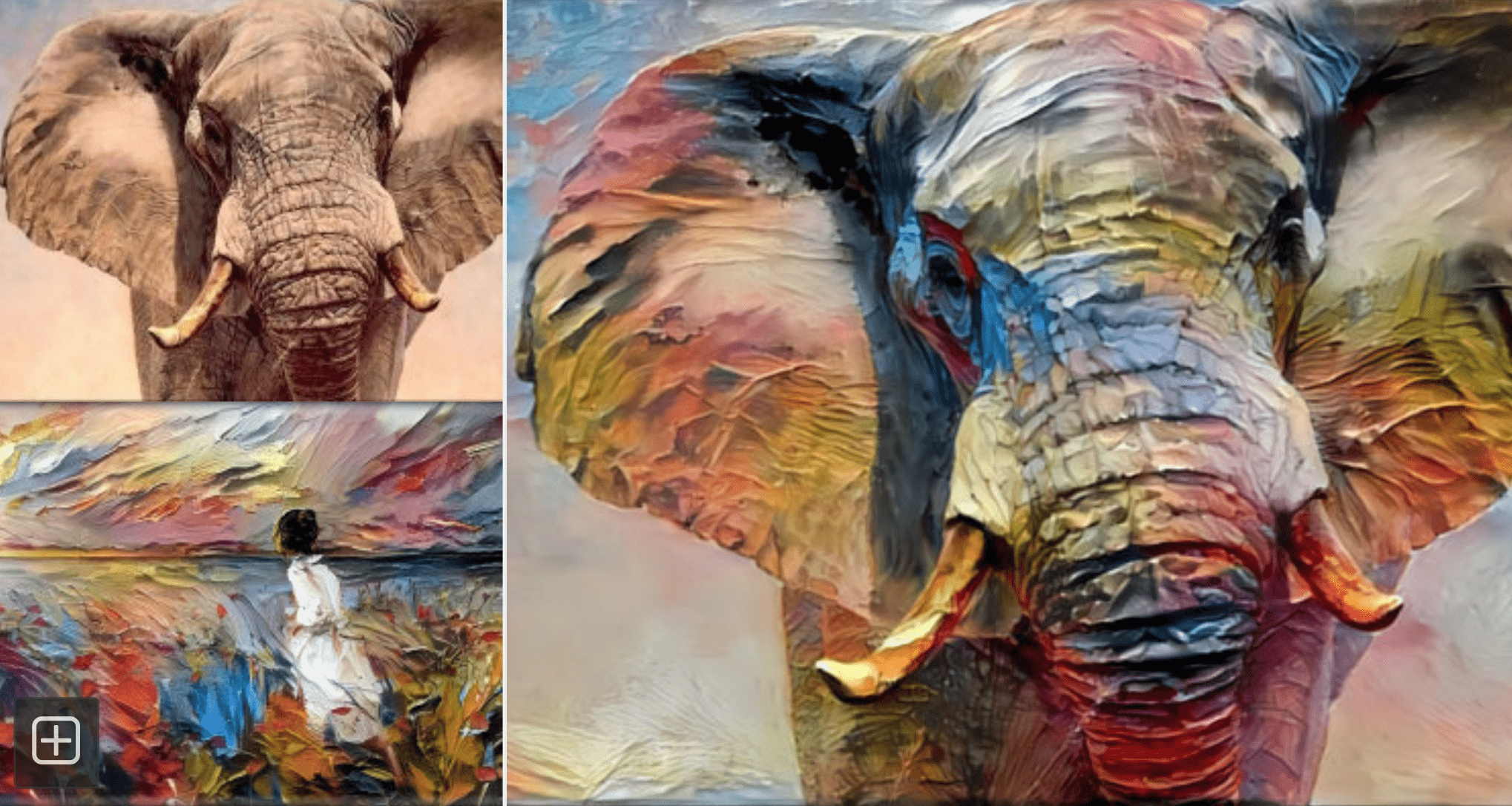# # GAN入门，有这篇就够了🎉🎉🎉GAN有两个神经网络🕸🕸。

GAN的目标

## # 训练思路📕

"抽"的这个动作相当于在分布$P_{data}(x)$里采样， 能够抽到到$\{x_1,x_2,...,x_n\}$, 也就是 $P_{data}(x_1),P_{data}(x_2),...,P_{data}(x_n)$的概率很高。生成器训练的目标是让$P_{G}(x)$$P_{data}(x)$越接近越好。因此我们希望$P_{G}(x_1; \theta),P_{G}(x_2; \theta),...,P_{G}(x_n; \theta)$ 中的每一个概率都很高, 换句话说就是想要$\prod_{k=1}^n P_{G}(x_k; \theta)$ 的值越大越好。

$x_1$被抽到了,那么一定是$P_{data}(x_1)$的概率高才会被抽到。

$\theta^* = \arg \max_{\theta} \prod_{k=1}^n P_{G}(x_k; \theta)$

$\arg \max_{\theta} \prod_{k=1}^n P_{G}(x_k; \theta) = \arg \min_{\theta} [KL(P_{data}||P_{G})]$

$\theta^* = \arg \min_{\theta} [KL(P_{data}||P_{G})]$

$\arg \max_{\theta} \prod_{k=1}^n P_{G}(x_k; \theta) = \arg \max_{\theta} \log \prod_{k=1}^n P_{G}(x_k; \theta)$

$= \arg \max_{\theta} \sum_{k=1}^n \log P_{G}(x_k; \theta)$, 由于$\{x_1,x_2,...,x_n\}$都来自于$P_{data}(x)$ 这个分布, 因此:

$\approx \arg \max_{\theta} \mathbb{E}_{x \sim P_{data}(x)}[\log P_{G}(x; \theta)]$

$= \arg \max_{\theta} [\int_x P_{data}(x)\log P_{G}(x;\theta)dx]$ 下一步减一个常数，对找$\theta ^*$没有影响。

$= \arg \max_{\theta} [\int_x P_{data}(x)\log P_{G}(x;\theta)dx - \int_x P_{data}(x)\log P_{data}(x)dx]$

$= \arg \max_{\theta} [\int_x P_{data}(x)\log \frac{P_{G}(x;\theta)}{P_{data}(x)}dx]$

$= \arg \max_{\theta} [- KL(P_{data}||P_{G})]$

$= \arg \min_{\theta} [KL(P_{data}||P_{G})]$

## # 具体步骤

Tip

### # 确认目的

$G^* = \arg \min_{G} Div(P_{G}, P_{data})$

### # 训练判别器

$V = \mathbb{E}_{x \sim P_{data}}[\log D(x)] + \mathbb{E}_{x \sim P_{G}}[\log (1-D(x))]$

$V$的表达式可以这样理解，在训练判别器计算分类器输出时：

1. 当图片$x$来自玲爱数据集时，$x$带入$\mathbb{E}_{x \sim P_{data}}[\log D(x)]$计算。
2. 当图片$x$来自生成器数据集时，$x$带入$\mathbb{E}_{x \sim P_{G}}[\log (1-D(x))]$计算。

$D^* = \arg \max_{D}V(D,G)$

• 给玲爱的画高分，即让$D(x)$的值越接近1越好;
• 给生成的画低分,即让$D(x)$的值越接近0越好。

$V(D,G) = \mathbb{E}_{x \sim P_{data}}[\log D(x)] + \mathbb{E}_{x \sim P_{G}}[\log (1-D(x))]$

$\tilde{V}=\frac{1}{m} \sum_{i=1}^{m} \log D\left(x^{i}\right)+\frac{1}{m} \sum_{i=1}^{m} \log \left(1-D\left(\tilde{x}^{i}\right)\right)$

• $x^i$来自玲爱数据集, 标签为1,
• $\tilde{x}^i$来自生成器数据集, 标签为0

## # 算法总结

GAN算法

• 初始化$D,G$的参数分别为$\theta_d, \theta_g$
• 循环训练:
• 训练$D$, $k$次:
• 从分布$P_{data}(x)$中随机采样$m$$\{x^1,x^2,...,x^m\}$
• 从已知分布$P_{prior}(z)$中随机采样$m$$\{z^1,z^2,...,z^m\}$
• 使用$\tilde{x}^i=G(z^i)$获得生成数据 $\{\tilde{x}^1, \tilde{x}^2,...,\tilde{x}^m\}$
• 更新$D$的参数$\theta_d$来最大化
• $\tilde{V}=\frac{1}{m} \sum_{i=1}^{m} \log D\left(x^{i}\right)+\frac{1}{m} \sum_{i=1}^{m} \log \left(1-D\left(\tilde{x}^{i}\right)\right)$
• $\theta_{d} \leftarrow \theta_{d}+\eta \nabla \tilde{V}\left(\theta_{d}\right)$
• 训练$G$, $1$次:
• 重新从已知分布$P_{prior}(z)$中随机采样$m$$\{z^1,z^2,...,z^m\}$
• 更新$G$的参数来最小化
• $\tilde{V}=\frac{1}{m} \sum_{i=1}^{m} \log D\left(x^{i}\right)+\frac{1}{m} \sum_{i=1}^{m} \log \left(1-D\left(\tilde{x}^{i}\right)\right)$
• $\theta_{g} \leftarrow \theta_{g}-\eta \nabla \tilde{V}\left(\theta_{g}\right)$

GAN在训练判别器时，生成器的参数固定不变；在训练生成器时，判别器的参数固定不变。 GAN的核心思想在这篇文章中算是介绍得差不多了。 当然最初的GAN也有很多不足之处，例如很难训练，不容易收敛等问题。因此又有很多人对原始的算法进行优化， 出现了各种各样衍生的GAN,我们以后有机会在聊一聊其他的GAN。

1. 首页图片来自JosieArt Deep Dream Generator
2. 国立台湾大李宏毅老师GAN课程 GAN Lecture 4 (2018): Basic Theory